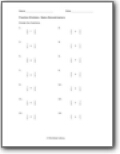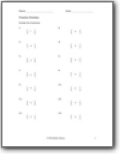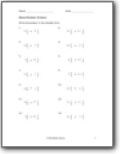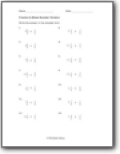# Fraction Division Worksheets

#### How to Divide Fractions

Dividing fractions can easily qualify as a child's worst math nightmare. The division is itself considered the most difficult math operation of all. Combining it with fractions makes it even more difficult for early-stage learners. However, in math, like everything can be mastered with practice, this can be fractional division can also be made easy. One trick of making fractional division easy is to take the reciprocal of the second fraction and exchange the division operation with the multiplication operation. After you flip the second fraction, there are two basic steps to find the quotient. The second step is to multiply the remaining numerators and you multiply the denominators. At that point you have completed the operation, you can simplify the fraction from there if you need to.###### Same Denominators

Divide the fractions that already share the same bottom number.###### Mixed Denominators

After you complete the division operation, write the answer in the simplest form. These fractions have both like and unlike denominators.###### Mixed Number Division

You will divide a mixed number by another mixed number. A go way to approach these problems is to start by converting everything to a simple fraction.###### Division Between Fractions & Mixed Numbers

One of the values is mixed numbers and the other value is a straight up fraction that you will divide into it.